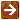﻿ Fee Calculations

#### Fee CalculationsUnless otherwise indicated, all calculations adhere to the standard rounding rules. For information on those rules, see Standard Rounding Rules.

As part of this process, the system determines how the fee calculation is to be made based upon the fee type specified in the KEMID Fee record.

If the fee type is T (Transactions), the system uses transaction records from the Transaction Archive table to calculate the fee.

If the fee rate definition code is C (Count), the system totals the number of the records that fit the selection criteria and have been posted since the last processing date for the fee.

If the rate definition code is V (Value), the system totals the income cash amount and/or principal cash amount of the records that fit the selection criteria and have been posted since the last processing date for the fee.

If the fee type is B (Balance), the system uses Current Tax Lot Balance or Holding History records to calculate the fee.

For records in which the fee rate definition code is C (Count), the system:

1.     Totals the number of units held by the records that fit the selection criteria.

2.     If the fee balance type is AU (Average Units), calculates the fee as the total of all holding units for records in which the month-end date is greater than the last processing date for the fee, divided by the number of records selected.

3.     If the fee balance type is MU (Month End Units), calculates the fee as the total of the holding units for all records in which the month-end date is the most recent month-end date.

4.     If the fee balance type is CU (Current Units), calculates the fee as the total of the current units held for all selected records.

For records in which the fee rate definition code is V (Value), the system:

1.     Examines the market value of the records that fit the selection criteria.

2.     If the fee balance type is AMV (Average Market Value), calculates the fee as the total of the holding market values for all Holding History records in which the month-end date is greater than the last processing date for the fee, divided by the number of records selected.

3.     If the fee balance type is MMV (Month End Market Value), calculates the fee as the total of the holding market values for all Holding History records in which the month-end date is the most recent month-end date.

4.     If the fee balance type is CMV (Current Market Value), calculates the fee as the total of all selected Current Tax Lot records.

The system then analyzes the appropriate KEMID records to determine whether a fee is to be charged and processes it appropriately. If a fee is to be charged, the system calculates fees against the total amount calculated for each KEMID according to the rules outlined above. The actual fee amount is calculated by adding together the results of these calculations:

Multiply the value of the total amount calculated that is less than or equal to the KEMID's fee break 1 by the KEMID's fee rate 1.

Multiply the value of the total amount calculated that is greater than the KEMID's fee break 1 and less than or equal to the KEMID's fee break 2 by the KEMID's fee rate 2.

Multiply the value of the total amount calculated that is greater than the KEMID's fee break 2 by the KEMID's fee rate 3.

The calculated fee is compared to the minimum fee amount charge. If it is less than the minimum fee amount charged, the system charges the minimum fee amount.

More: